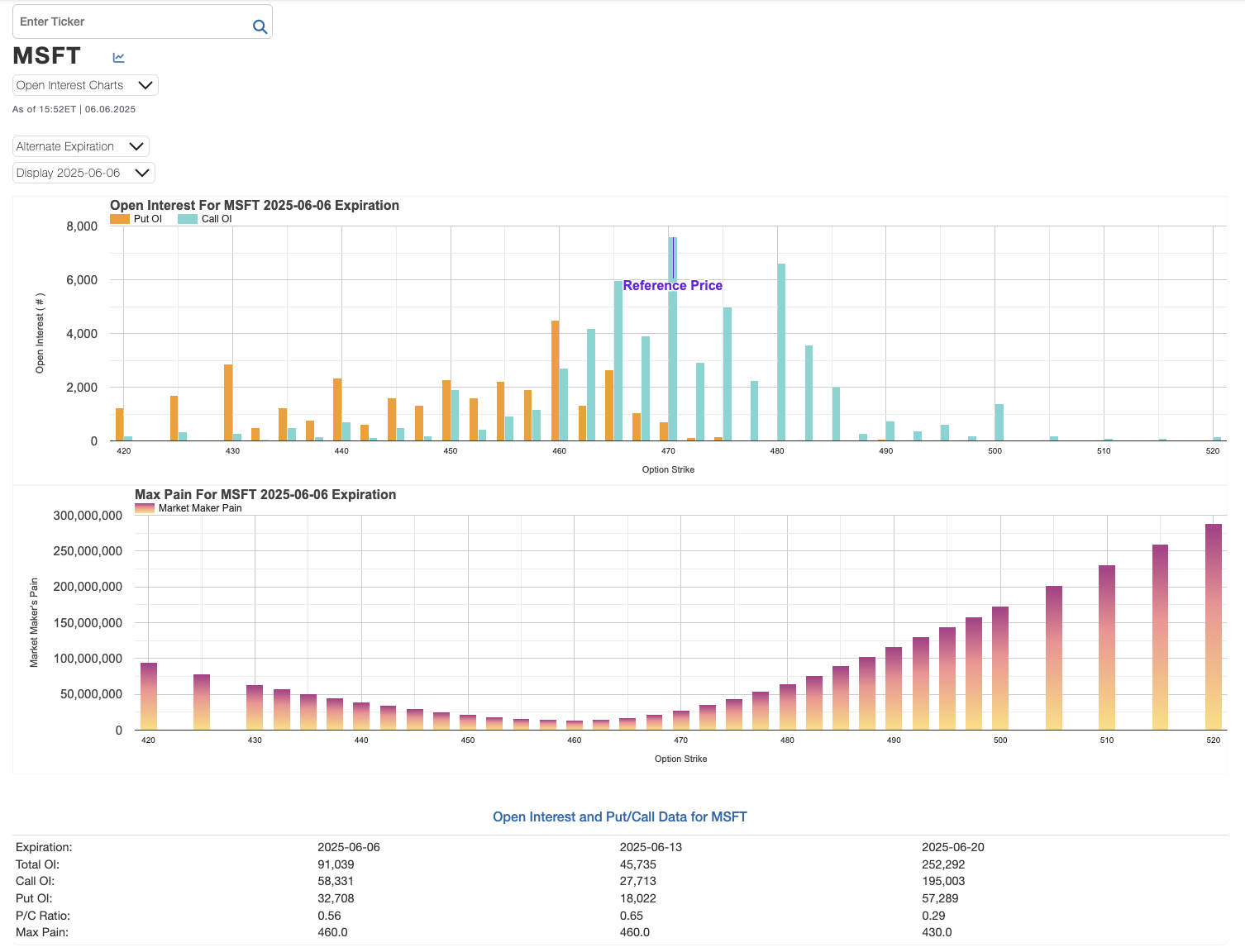Below is the Open Interest for Puts and Calls for the next three options expiration dates, along with the calculated Max Pain based on Open Interest. Open Interest data and Put/Call Ratios are shown below the charts.

Sign Out
Below is a sample of our Max Pain charts.###### ***Max Pain is calculated by summing the loss at each strike, i.e The sum of the intrinsic value * Open Interest.

Open Interest and Put/Call Data